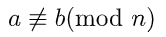安卓手机扫描二维码安装App

A. ﻿$2 \space \mathrm{mod} \space 7=5$﻿

B. ﻿$-20 \space \mathrm{mod} \space 6 =2$﻿

C. ﻿$9 = 16 (\mathrm{mod} \space 7)$﻿

D. ﻿$252 = 483 ( \mathrm{mod} \space 10)$﻿

﻿$n$﻿ 为正整数，﻿$a$﻿﻿$b$﻿ 为整数. 如果 ﻿$a$﻿﻿$b$﻿﻿$n$﻿ 除后余数相同，那么称 ﻿$a$﻿﻿$b$﻿﻿$n$﻿ 同余，记作

﻿$a \equiv b ( \mathrm{mod} \space n)$﻿ .

﻿$a$﻿﻿$b$﻿﻿$n$﻿ 除后余数不同，则称 ﻿$a$﻿﻿$b$﻿﻿$n$﻿ 不同余，记作﻿$\mathrm{mod}$﻿ 这个记号是德国数学家高斯（Gauss，1777－1855）发明的.苹果手机扫描二维码安装App